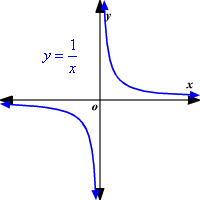# Parent Graphs

A parent graph is the graph of a relatively simple function. By transforming the function in various ways, the graph can be translated, reflected, or otherwise changed.

Below are some common parent graphs:

 Linear: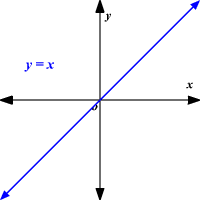Absolute Value: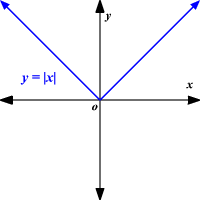Quadratic: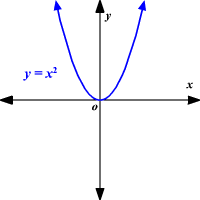Square Root: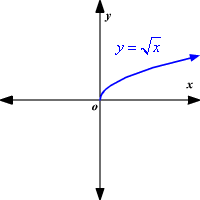Cubic: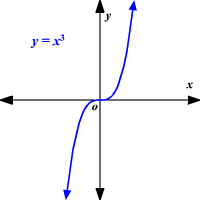Exponential: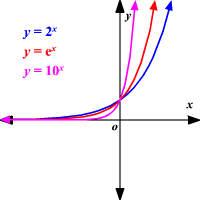Logarithmic: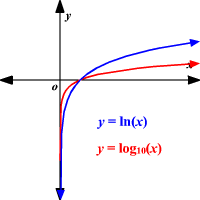Sine: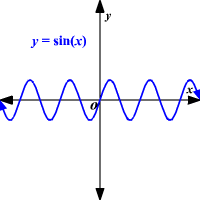Cosine: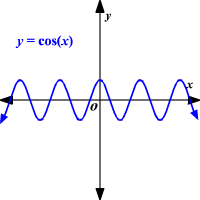Tangent: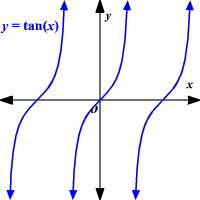Hyperbolic: